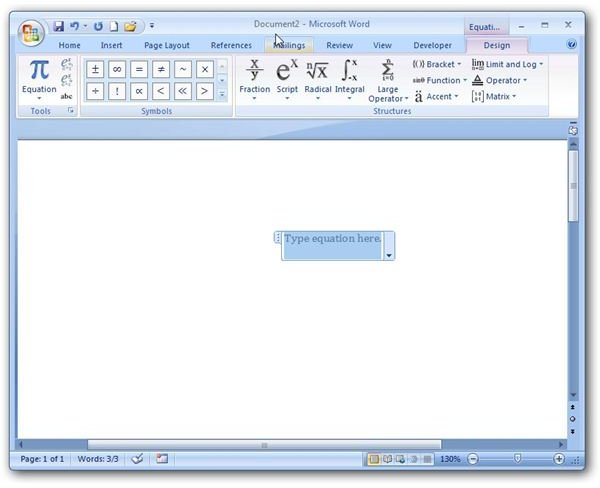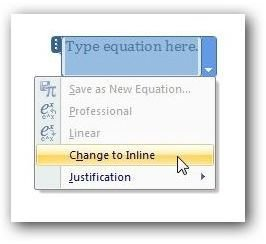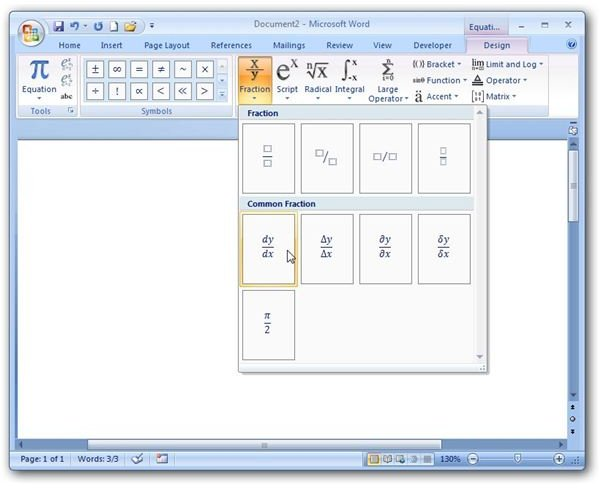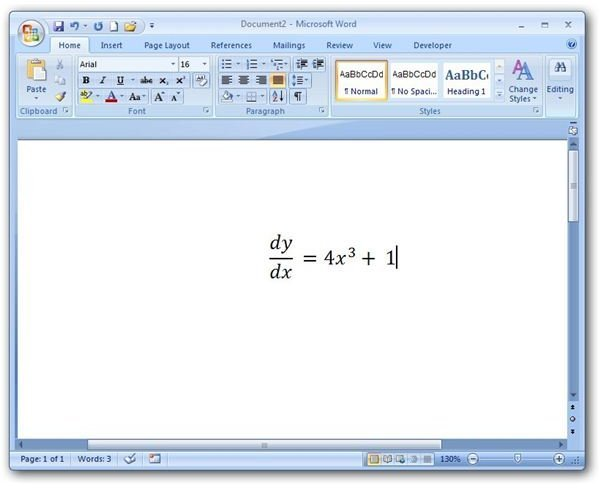# How to Insert Mathematical Equations and Symbols in MS Word 2007

Page content

## Mathematical Symbols and Word 2007

While there are a number of applications that specialize in creating documents that include a lot of mathematical symbols, these products can be both expensive and difficult to learn. However, word processors – including Microsoft Word – have come a long way in this regard over the past few years. Now, it’s quite possible that you can use Word 2007 for all of your works that include formulas, equations, and other mathematical content.

Accessing the Equation Editor

Step 1: To begin, place your cursor at the point in the document where you want to insert the mathematical content. Then, open the Insert tab on the Word ribbon and click Insert Equation. (Click any image for a larger view.)

Step 2: A new addition called Equation Tools should now be seen on the Word Ribbon. A Design tab will appear underneath this heading.Also, a box will be inserted into the document in which you can create and edit the mathematical formula. By default, this equation box will be in Display mode, meaning that it is centered on the next blank line of your document. If you would prefer to have the equation inserted as standard text, click on the arrow next to this box and select Change to Inline.Note that clicking anywhere else in the document will cause the equation box to become inactive. To make it active again, just click on the area with the text Type equation here. Also note that you can change the font and formatting of the equation in the same manner that you would for any other text.

Step 3: Using the tools on the Design tab, build your equation or formula. The first few times you use the equation editor, you might have to do a bit of hunting for some symbols, but most are in intuitive locations. For example, although differentials appear under the Integral tool, derivative forms are found under Fraction.Step 4: After you’ve completed the design of your equation or formula, click anywhere outside the equation box and you’ll be returned to the main Word document with the addition in place. If, at anytime, you need to edit the mathematical content, hover the mouse pointer over the area and click on the equation. Once again, you’ll be in the editor and can make any desired changes.Additional Resources: If you’re looking for more guides and how-to articles, be sure to browse through the other items in Bright Hub’s collection of Microsoft Word tutorials.